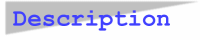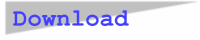## Curvit for WindowsCurVit estimates regression parameters for linear and for linearly transformable functions. It computes four goodness-of-fit criteria viz. RMSE = Root Mean Squared Error, MAD = Mean Absolute Deviation, MAPE = Mean Absolute Percentage Error, and MPE = Mean Percentage Error or Bias. Up to ten forecast values may be calculated for the regression function of the user's choice. Input and fitted data are plotted in a line graph. The test data file SAMPLE21.DAT contains 21 datapoints.